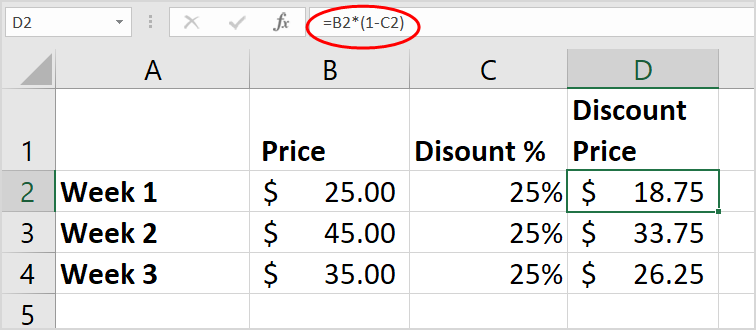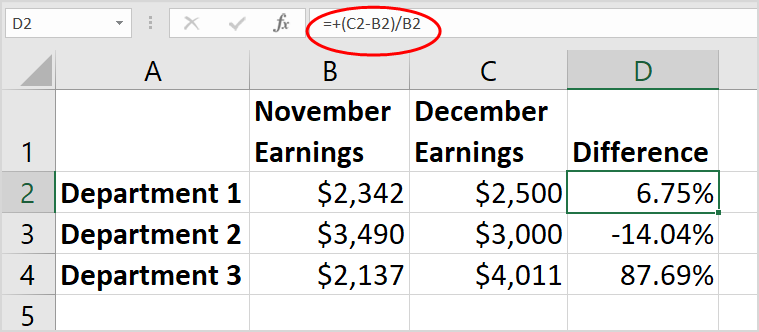# Excel formula: how to add percentage in Excel (4 Easiest Ways)

July 21, 2022
23.4K Views
0

A free Office suite fully compatible with Microsoft OfficeYou can compute percentages in Excel in a variety of ways. Excel may be used to determine the % of right answers on a test, discount prices using various percent assumptions, and calculate the percent change between two numbers, for example. In Excel, calculating a percentage is a simple two-step procedure. The percent formula is built in a cell once the cell is formatted to indicate the value is a percent.

Format values as percentage in excel online, 2016 and 2019

1.In Excel, you must use the Percentage format to display a value as a percentage. Select the cells to format, then click the Percent Style (percent) button in the Number group on the Home tab of the ribbon. The decimal place can then be increased (or decreased) as desired. (For further detail, see the section on rounding difficulties below.)2. The underlying value in Excel is always saved in decimal format. So, even if you've used numerical formatting to show something as a percentage (10%), it's still just that—formatting, or a symbolic representation of the underlying value. Excel always calculates the underlying value, which is a decimal number (0.1). Select the cell, press Ctrl + 1, then look in the Sample box under the General category to double-check the underlying value.There are few things that you need to keep in mind while formatting percentages:

Formatting existing values in excel

When you use percentage formatting on a cell with a number already in it, Excel multiplies it by 100 and adds the percent sign at the end. So, if you write 10 into cell A2 and then apply the percentage number format, Excel will multiply your number by 100 to display it as a percentage (remember that 1 percent is one part of one hundred), so the cell will reflect 1000 percent, not 10%. You may get around this by first calculating your numbers as percentages.

If you write the formula =10/100 in cell A2, Excel will return 0.1 as the result. When you format that decimal as a percentage, the number will appear as 10%, as you would anticipate. You may also simply put the decimal form of the value into the cell—for example, type 0.1 and then apply percentage format.

Rounding issues in excel

Occasionally, the number you see in a cell (for example, 10%) does not match the amount you anticipated to see (e.g., 9.75 percent ). Increase the decimal places to view the real percentage in the cell rather than a rounded version. Excel always performs computations based on the underlying number.

How to calculate percentage in excel

1. As with any Excel formula, begin by entering an equal sign (=) in the cell where you want your result, then the rest of the calculation. =part/total is the fundamental formula for determining a percentage.

Actual Points/Possible Points = Grade Percentage in the case below:2. Assume you wish to cut a specific amount by 25%, as though you're trying to apply a discount. The formula will be =Price*1-Discount percent in this case. (Think of the 1 as a placeholder for 100%.)3. Simply replace the negative sign in the calculation above with a plus sign to boost the amount by 25%.

The following example is a little trickier. Let's say your department's earnings were \$2,342 in November and \$2,500 in December, and you're looking for the % change in earnings. Divide the difference between December and November profits (\$158) by the value of November earnings (\$2,342) to get the answer.Note: This was an attempt to show you how to add percentage in excel online, 2016 and 2019 versions, be it on windows or Mac.

You just need to have a little understanding of how and which way things work and you are good to go. With having this basic knowledge or information of how to use it, you can also access and use different other options on excel or spreadsheet. Also, it is very similar to Word or Document. So, in a way, if you learn one thing, like Excel, you can automatically learn how to use Word as well because both of them are very similar in so many ways. If you want to know more about WPS Office, you can download WPS Office to access, Word, Excel, PowerPoint for free.